## Sunday, February 27, 2011

### OCW-18.443: problem-set#4

My solutions to 4th problem set in MIT-Statistics for Applications course.

You should read the handout given with this assignment as same material is not covered in the text book.

1. We can simply use the known(derived in exp-8.4.3 of "Mathematical Statistics and Data Analysis") formulae. Here is the R-terminal interaction...
> data = c(0.312,0.238,0.446,0.968,0.576,0.471,0.596)
>
> estimated_variance = var(data)
> estimated_mean = mean(data)
>
> estimated_lambda = estimated_mean / estimated_variance
> estimated_lambda
 9.089924
>
> estimated_alpha = (estimated_mean * estimated_mean)/estimated_variance
> estimated_alpha
 4.683908
>
>

2. Let T(X) be an unbiased estimator of $p^2$
Then E[T(X)] = $p^2$
=> T(0)P(X=0) + T(1)P(X=1) + T(2)P(X=2) = $p^2$
=> ${(1-p)}^2$T(0) + 2p(1-p)T(1) + $p^2$T(2) = $p^2$
...
...
=> $p^2$(T(0) - 2T(1) + T(2) - 1) + 2p(T(1) - T(0)) + T(0) = 0

For above to be true for all values of p in [0,1], we have
T(0) - 2T(1) + T(2) - 1 = 0
T(1) - T(0) = 0
T(0) = 0

On solving above we get
T(0) = 0
T(1) = 0
T(2) = 1

This is the unbiased estimator of $p^2$. Most surprising part of above estimator is that it estimates $p^2$ to be 0 even if X = 1 that is there was one success(and hence p should not be 0)

3.4.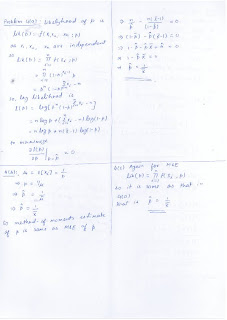## Saturday, February 26, 2011

### OCW-18.443: problem-set#3

My solutions to 3rd problem set in MIT-Statistics for Applications course.

Most of the solutions are done using R(version 2.12.1).

Note: In this problem set, if you don't understand the meaning of y-on-x, x-on-y and bfsd regression, then you should read the handouts given for this assignment.

1(a)
y - distance
x - year
years are constant and also not measured using some instrument(and hence no chances of error), so clearly year is not a random variable. Hence the most suitable regression here is y-on-x.

1(b) Here is the R-terminal interaction.
>
> year = c(1952,1956,1960,1964,1968,1972,1976,1980,1984)
> distance = c(758.3,784.4,813.6,808.5,891.9,825.7,835.9,855.6,855.6)
>
> plot(year,distance) # draw distance(y-axis) vs year(x-axis) plot
>>
> #clearly (1968,891.9) is an outlier, otherwise it looks close to linear
>
> linmodel = lm(distance ~ year) #create the linear model
> residuals = resid(linmodel); residuals #find and print the residuals
1 2 3 4 5 6 7
-22.893333 -7.870000 10.253333 -5.923333 66.400000 -10.876667 -11.753333
8 9
-3.130000 -14.206667
>
> plot(year, residuals) #plot residuals vs year
>1(c) I notice that 7 out of 9 residuals are negative. And, actually there seems to be a pattern.

2(a)
y - power output
x - wind speed

If we do y-on-x regression, prediction for power output at sufficiently small speeds comes negative which is not possible. Also, no one will be interested in predicting wind speed from power output(as naturally power is being produced by wind and not viceversa) :).

2(b)
First, let us find the correlation coefficients for all 10 values using R. Here it is
>
> output = c(0.123,0.558,1.194,1.582,1.822,1.800,2.166,2.303,2.386,2.236)
>
> speed = c(2.45,3.05,4.10,5.00,6.00,7.00,8.15,9.10,9.70,10.00)
>
> cor(output,speed)
 0.9419852
>

Now, let us find the correlation for 7 values where windspeeds vary from 3 to 9.1 mph.
>
> cor(output[2:8],speed[2:8])
 0.951478
>

Now, let us do the y-on-x regression for the 7 values.
>
> speed = speed[2:8]
> output = output[2:8]
>
> linmodel = lm(output ~ speed) #build the model
> coeffs = coefficients(linmodel)
>
> #Plot the output vs speed along with fitted line
> plot(speed,output,xlab="Wind Speed",ylab="Power Output")
> abline(coeffs,coeffs)
>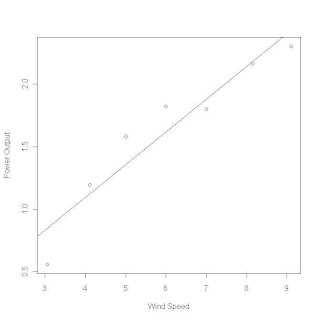3.
Again, I solved it using R. Here is R-terminal interaction.

> height = c(5000,10000,15000,20000,25000,30000,35000,40000,45000,50000,
55000,60000,70000,80000,90000,100000)
>
> pressure = c(632.50,522.70,429.00,349.50,282.40,226.10,179.30,141.20,
111.10,87.80,68.90,54.20,33.70,21.00,13.20,8.36)
>
> plot(height,pressure)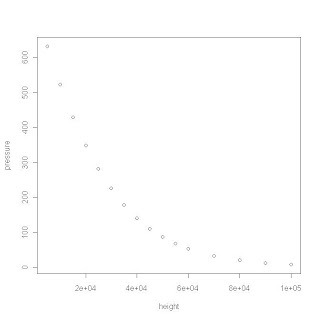> #clearly it does not look linear so we can expect some pattern in residuals
>
>
> linmodel = lm(pressure ~ height)
>
> coeffs = coefficients(linmodel)
> coeffs #Intercept
(Intercept)
471.6105
> coeffs #slope
height
-0.006006586
>
> cor(height,pressure) #correlation
 -0.885868
>
> residuals = resid(linmodel)
>
> residuals
1 2 3 4 5 6 7
190.922430 111.155362 47.488294 -1.978775 -39.045843 -65.312911 -82.079980
8 9 10 11 12 13 14
-90.147048 -90.214116 -83.481185 -72.348253 -57.015322 -17.449458 29.916405
15 16
82.182268 137.408132
>
> plot(height,residuals)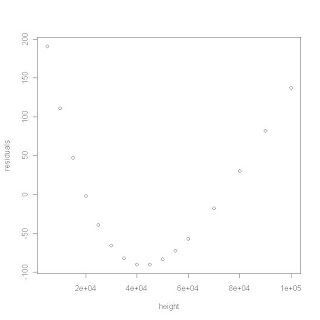Clearly residuals vs height plot shows a pattern and as expected data does not fit linear model.

4.
We are using height, pressure from problem#3
>
> squared_height = height * height
> squared_height
 2.500e+07 1.000e+08 2.250e+08 4.000e+08 6.250e+08 9.000e+08 1.225e+09
 1.600e+09 2.025e+09 2.500e+09 3.025e+09 3.600e+09 4.900e+09 6.400e+09
 8.100e+09 1.000e+10
>
> plot(squared_height,pressure)> #clearly this does not look linear either, so we can expect pattern in residuals
>
> linmodel = lm(pressure ~ squared_height)
>
> coeffs = coefficients(linmodel)
> coeffs #Intercept
(Intercept)
332.7193
> coeffs #slope
squared_height
-4.737236e-08
>
> cor(squared_height,pressure) #correlation
 -0.7394696
>
> residuals = resid(linmodel)
>
> residuals
1 2 3 4 5 6
300.965031 194.717958 106.939504 35.729667 -20.711551 -63.984150
7 8 9 10 11 12
-95.388132 -115.723495 -125.690240 -126.488366 -120.517875 -107.978765
13 14 15 16
-66.894691 -8.536143 64.196877 149.364370
>
> plot(squared_height,residuals)Clearly residuals vs height plot shows a pattern.

5.
Again, the R-terminal interaction is here...
> ln_pressure = log(pressure)
> ln_pressure
 6.449680 6.259008 6.061457 5.856504 5.643325 5.420977 5.189060 4.950177
 4.710431 4.475062 4.232656 3.992681 3.517498 3.044522 2.580217 2.123458
>
> plot(height,ln_pressure)> #clearly its linear, so there shouldn't be any pattern in residuals
>
> linmodel = lm(ln_pressure ~ height)
>
> coeffs = coefficients(linmodel)
> coeffs #Intercept
(Intercept)
6.76613
> coeffs #slope
height
-4.623476e-05
>
> cor(ln_pressure,height) #correlation
 -0.9996577
>
> residuals = resid(linmodel)
> residuals
1 2 3 4 5
-0.0852764784 -0.0447752103 -0.0111521741 0.0150682717 0.0330630186
6 7 8 9 10
0.0418896953 0.0411464997 0.0334372471 0.0248644217 0.0206690287
11 12 13 14 15
0.0094375091 0.0006360425 -0.0121994225 -0.0228272162 -0.0247852183
16
-0.0191960148
>
> plot(height,residuals)Though plot shows some pattern but residual absolute values are tiny compared to those in problem#3 and problem#4. However to be sure that our model is correct. Let us plot the data points and fitted line together and see that it fits well
>
> plot(height,ln_pressure)
> abline(6.76613,-0.00004623476)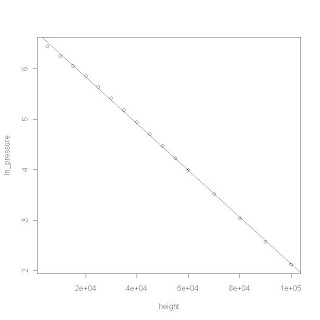## Thursday, February 24, 2011

### OCW-18.443: problem-set#1

My solutions to 1st problem set in MIT-Statistics for Applications course.

Some of the solutions are done using R(version 2.12.1).

Also, they use following function that prints out the confidence interval for mean given the sample mean, estimated standard deviation, percentage and size of the sample.
n_conf_interval_mean = function(mean, sd, percentage, size) {  error = qnorm((percentage/100 + 1)/2)*sd/sqrt(size)  left = mean - error  right = mean + error  sprintf("Required interval is (%f,%f).",left,right)}

1(a) Sum of independent normal random variables is another normal random variable. So sample mean(Xbar) is also normal random variable with
E[Xbar] = 0 and
Var(Xbar) = 1/n = 1/49

So, (Xbar - E[Xbar])/$\sqrt{Var(Xbar)}$ is approximately N(0,1). That is, 7Xbar is N(0,1).

Now, P(|Xbar| < c) = 0.8
=> P(-c < Xbar < c) = 0.8
=> P(-7c < 7Xbar < 7c) = 0.8
=> P(-7c < Z < 7c) = 0.8 , where Z is standard normal random variable
=> 2F(7c) - 1 = 0.8 , where F is CDF of standard normal random variable
=> F(7c) = 0.9

From the table of CDF of standard normal distribution we can see that F(1.28) = .8997
So approximately 7c = 1.28
=> c = 0.183

1(b) T follows a t distribution with 4 degrees of freedom.

(i) P(|T| < t0) = 0.9
=> P(-t0 < T < t0) = 0.9
=> 2P(T < t0) - 1 = 0.9
=> P(T < t0) = 0.95
by looking at the quantiles of t-distribution table, we can check that t0 = 2.132

(ii) P(T > t0) = 0.05
=> 1 - P(T < t0) = 0.05
=> P(T < t0) = 0.95
so again, t0 = 2.132

2. We will solve this with R. Here is the R terminal interaction:
>
> mean(data) #2(a)
 6.6766
>
> sd(data) #2(b)
 0.006202688
>
> (6.6766 - 6.1)/0.006202688 #2(c)
 92.9597
>
> n_conf_interval_mean(6.6766,0.006202688,95,7) #2(d)
 "Required interval is (6.672005,6.681195)."
>

3(a).
$\sigma^2$ can be estimated by
$S^2$ = $\frac{1}{n-1}\sum_{i=1}^n {(X_i - Xbar)}^2$

Also, $\frac{(n-1)S^2}{\sigma^2}$ has chi-square distribution with n-1 degrees of freedom.

Let $F_m(\alpha)$ denote the point beyond which the chi-square distribution with m degrees of freedom has probability $\alpha$. That is, $F_m(\alpha)$ is (1-$\alpha$)th quantile of chi-square distribution with m degrees of freedom.
Then it can be derived(a similar derivation is done in Example-8.5.6 in book " Mathematical Statistics and Data Analysis ") that

(1-$\alpha$)100 % confidence interval for $\sigma^2$ is
($\frac{(n-1)S^2}{F_{n-1}(\alpha/2)}$ , $\frac{(n-1)S^2}{F_{n-1}(1 - \alpha/2)}$)

Required caculations are done with R. Here is the R-terminal interaction

> data
 6.6729 6.6735 6.6873 6.6699 6.6742 6.6830 6.6754
>
> S_square = var(data)
> S_square
 3.847333e-05
>
> n = 7
>
> alpha = 1 - 95/100
>
> left = (n-1)*S_square/qchisq(0.975,6)
> right = (n-1)*S_square/qchisq(0.025,6)
>
> sprintf("95 percent confidence interval for sigma-square is (%f,%f)",left,ri$ "95 percent confidence interval for sigma-square is (0.000016,0.000187)" > > sprintf("95 percent confidence interval for sigma is (%f,%f)",sqrt(left),sqrt(right))  "95 percent confidence interval for sigma is (0.003997,0.013659)" > 3(b) Clearly 0.0005, 0.0007, 0.0006 are withing the confidence interval for$\sigma\$

4.
Again, we will use R to solve this. Here is the R-terminal interaction
> n_conf_interval_mean(98.41,0.77,95,26) #4(a)
 "Required interval is (98.114027,98.705973)."
>
> n_conf_interval_mean(98.10,0.72,95,122) #4(b)
 "Required interval is (97.972238,98.227762)."
>

(c) Yes, the intervals overlap. Yes, 95% confidence interval for women's temperature data contains 98.6

5. Here is the R-terminal interaction
> x <- rexp(25)
> shapiro.test(x)

Shapiro-Wilk normality test

data: x
W = 0.7748, p-value = 8.875e-05

> y <- runif(200)
> shapiro.test(y)

Shapiro-Wilk normality test

data: y
W = 0.9521, p-value = 2.999e-06

> z <- rnorm(500)
> shapiro.test(z)

Shapiro-Wilk normality test

data: z
W = 0.9982, p-value = 0.8914

>